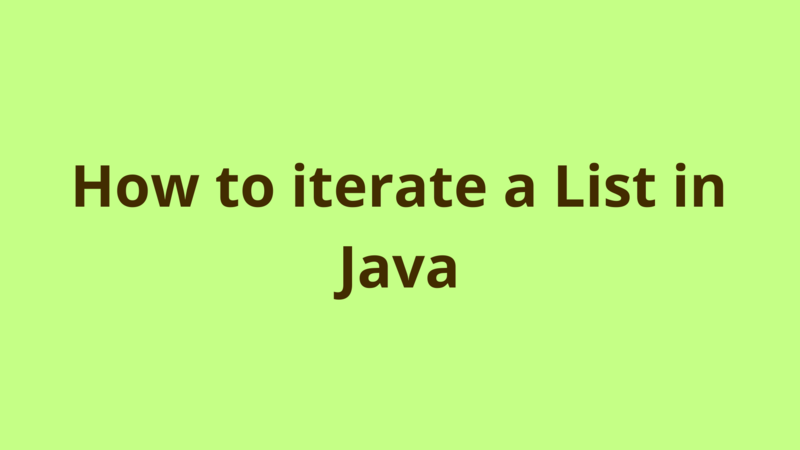## Introduction

This tutorial shows several ways for iterating a List in Java.

## 1- Typical For loop

Using a typical For loop, you can iterate a List as the following:

``````private static void iterateListForLoop(List<String> lstInput)
{
for(int i=0; i<lstInput.size(); i++)
{
System.out.println(lstInput.get(i));
}
}
``````

## 2- Enhanced For loop

Since JDK 5.0, you can iterate a List using an enhanced For loop which works as the following:

``````private static void iterateListForEach(List<String> lstInput)
{
for(String input : lstInput)
{
System.out.println(input);
}
}
``````

## 3- Typical While loop

Using a typical while loop, you can iterate a List as the following:

``````private static void iterateListWhileLoop(List<String> lstInput)
{
int i=0;
while(i<lstInput.size())
{
System.out.println(lstInput.get(i));
i++;
}
}
``````

## 4- Iterator

Collections in Java can be iterated through Iterator as the following:

``````private static void iterateListIterator(List<String> lstInput)
{
for(Iterator<String> iter = lstInput.iterator(); iter.hasNext();)
{
System.out.println(iter.next());
}
}
``````

## 5- Java 8

With Java 8, you can iterate a List in one line using forEach() method as the following:

``````private static void iterateListJava8(List<String> lstInput)
{
lstInput.forEach((name) -> System.out.println(name));
}
``````

## Summary

This tutorial shows several ways for iterating a List in Java.

## Next Steps

If you're interested in learning more about the basics of Java, coding, and software development, check out our Coding Essentials Guidebook for Developers, where we cover the essential languages, concepts, and tools that you'll need to become a professional developer.

Thanks and happy coding! We hope you enjoyed this article. If you have any questions or comments, feel free to reach out to jacob@initialcommit.io.